# Using R for the examples in “Beyond Bar and Line Graphs: Time for a New Data Presentation Paradigm’

### What is this?

Here I show how we can use R to reproduce the plots described in:

Weissgerber TL, Milic NM, Winham SJ, Garovic VD. Beyond Bar and Line Graphs: Time for a New Data Presentation Paradigm. PLOS Biology, 2015:13. http://journals.plos.org/plosbiology/article?id=10.1371/journal.pbio.1002128

I have taken the Excel spreadsheet files included with the PLOS Biology publication and written R code to read the sample data from the Excel files, and recreate the plots in the Excel files. Much of the text here is also adapted from the how-to notes in the Excel files. To run the code in this document you’ll need to install, in addition to R, the following packages readxl, tidyr, dplyr, and ggplot2. This can be done with this line at the R prompt: install.packages(c("readxl", "tidyr", "dplyr", "ggplot2")). The full details of packages and version used here can be found at the end of this document. You’ll also need the Excel files that accompany the paper (inlcuded here and available at the URL above for the paper).

I used RStudio to write the code, and made extensive use of the View() function to identify the exact rows and columns of the spreadsheets that had the sample data. To run the code below, I recommend using RStudio, downloading the GitHub repository that contains this file and the Excel files (zip file), and opening the new-data-presentation-paradigm-using-r.Rproj file, which will open this source file and set the working directory to the location of this file on your computer.

### Why bother?

The Weissgerber et al. paper makes a good argument for shifting the default data visualisation from bar plots to dot plots that show more information about the data. They provide an Excel spreadsheet with a template for producing their preferred plots, and instructions for using GraphPad PRISM. But there are no instructions for making their dot plots using free and open source software, which limits the usefulness of the paper. R is a good free and open source choice to implement these plots because it produces high quality visualisations with relative ease. Making these plots in R also solves a few problems in the Excel spreadsheets provided by Weissgerber et al. For example:

• The Excel spreadsheets are limited to 15-20 observations, (more than that requires some fiddling about), but the only limitation using R is the memory of your computer (so thousands to millions of observations should be no problem for most people).
• Some of the plots in the Excel sheet don’t have axis tick labels or axis labels. These are taken care of automatically with the R code below.
• Note that the plotting functions below compute the medians for us, so we don’t need any behind-the-scenes stuff like in the Excel spreadsheet.
• In the chunks below you can very easily change the axis labels by changing the text between the double quote marks in the xlab and ylab fields.

There are a few other demonstrations of R programming relevant to the Weissgerber et al paper:

The main limitation of these is that they are not very close to the plots and data contained in the publication. The code below aims for a very close reproduction the supplementary materials for the Weissgerber et al paper. This high fidility replication makes it easier to compare the Excel methods to the R methods. Hopefully it will be useful if you’re looking to get started in using R for plotting.

The source of this document is located at https://github.com/benmarwick/new-data-presentation-paradigm-using-r and the rendered version can be viewed online at To see the plots click here: https://cdn.rawgit.com/benmarwick/new-data-presentation-paradigm-using-r/master/Weissgerber_et_al_supplementary_plots.html

## Interpreting the plots

The short horizontal black lines in the plots show the median values. The mean should not be shown for data that are analyzed non-parametrically, as these data do not meet the distributional assumptions required to calculate the mean. If your data meet the assumptions required for parametric testing the graphs can be changed to show the mean instead of the median. To use the mean with the code below, simply replace “median” with “mean” where it occurs in the chunks below. Open circles show measurements for each participant or observation.

## Independent data, points not jittered (no overlapping points)

Use these next few chunks of code to create scatterplots for independent data in two to five groups, when there are no overlapping points within any group. Independent data means that the variable of interest is measured one time in each subject, and subjects are not related to each other. If your data do not meet this criteria, see the further below for paired or non-independent data. Overlapping points means that two subjects have values that are so close that they will overlap on the graph and you will not be able to see both points clearly. If your data have overlapping points, use the code in the “Points Jittered” section below.

First step is to read in the data from Excel spreadsheet (which needs to be in the same folder as this source file, or else you need to specify the full path to the file on your computer), then extract the specific rows and columns that contain the data to be plotted. The table below shows the format of the data in the Excel sheet. This chunk below can be adapted to read data from any Excel file, just change the file name and adapt the subsetting line so that it captures the relevant rows and columns in the Excel file.

library(readxl)
# read in data from PLOS Biology article supplementary materials
independent_data <- read_excel("journal.pbio.1002128.s002.XLSX", sheet = 1)
# subset just groups 1-5 from the 'No overlapping points' sheet
independent_data <- independent_data[15:30,2:6]
# assign column names
names(independent_data) <- independent_data[1, ]
# remove first row with column names
independent_data <- independent_data[-1, ]
kable(independent_data)
Group 1 Group 2 Group 3 Group 4 Group 5
16 5 7 9 42 2
17 3 3 7 2 0
18 6 9 10 5 3
19 8 10 12 55 5
20 10 33 14 9 7
21 13 15 17 12 10
22 1 18 20 15 13
23 4 6 40 3 1
24 18 20 22 NA 15
25 4 30 35 NA 1
26 7 NA 42 NA 4
27 9 NA 13 NA 6
28 14 NA NA NA 11
29 15 NA NA NA 12
30 17 NA NA NA 14

An important step is reshaping the data from their current wide format to a more tidy long format. Long formats are most useful for plotting and statistical analysis in R. Here’s what the data look like in the long format:

# reshape for plotting
library(tidyr)
independent_data_long <- gather(independent_data, group, value, Group 1:Group 5, convert = TRUE)
kable(independent_data_long)
group value
Group 1 5
Group 1 3
Group 1 6
Group 1 8
Group 1 10
Group 1 13
Group 1 1
Group 1 4
Group 1 18
Group 1 4
Group 1 7
Group 1 9
Group 1 14
Group 1 15
Group 1 17
Group 2 7
Group 2 3
Group 2 9
Group 2 10
Group 2 33
Group 2 15
Group 2 18
Group 2 6
Group 2 20
Group 2 30
Group 2 NA
Group 2 NA
Group 2 NA
Group 2 NA
Group 2 NA
Group 3 9
Group 3 7
Group 3 10
Group 3 12
Group 3 14
Group 3 17
Group 3 20
Group 3 40
Group 3 22
Group 3 35
Group 3 42
Group 3 13
Group 3 NA
Group 3 NA
Group 3 NA
Group 4 42
Group 4 2
Group 4 5
Group 4 55
Group 4 9
Group 4 12
Group 4 15
Group 4 3
Group 4 NA
Group 4 NA
Group 4 NA
Group 4 NA
Group 4 NA
Group 4 NA
Group 4 NA
Group 5 2
Group 5 0
Group 5 3
Group 5 5
Group 5 7
Group 5 10
Group 5 13
Group 5 1
Group 5 15
Group 5 1
Group 5 4
Group 5 6
Group 5 11
Group 5 12
Group 5 14

Now we are ready to plot, starting with subsetting just groups 1 and and 2 from the long data frame. Open circles show measurements for each participant or observation.

# plot
library(ggplot2)
library(dplyr)
# subset groups 1 & 2
independent_data_long_groups_1_and_2 <- independent_data_long %>%
filter(group %in% c("Group 1", "Group 2"))
# plot
ggplot(independent_data_long_groups_1_and_2, aes(group, as.numeric(value))) +
geom_point(shape = 1, size = 4) +
stat_summary(fun.y = median, fun.ymin = median, fun.ymax = median, geom = "crossbar", width = 0.2, size = 1) +
xlab("") +
ylab("Measurement (units)") +
theme_minimal(base_size = 16)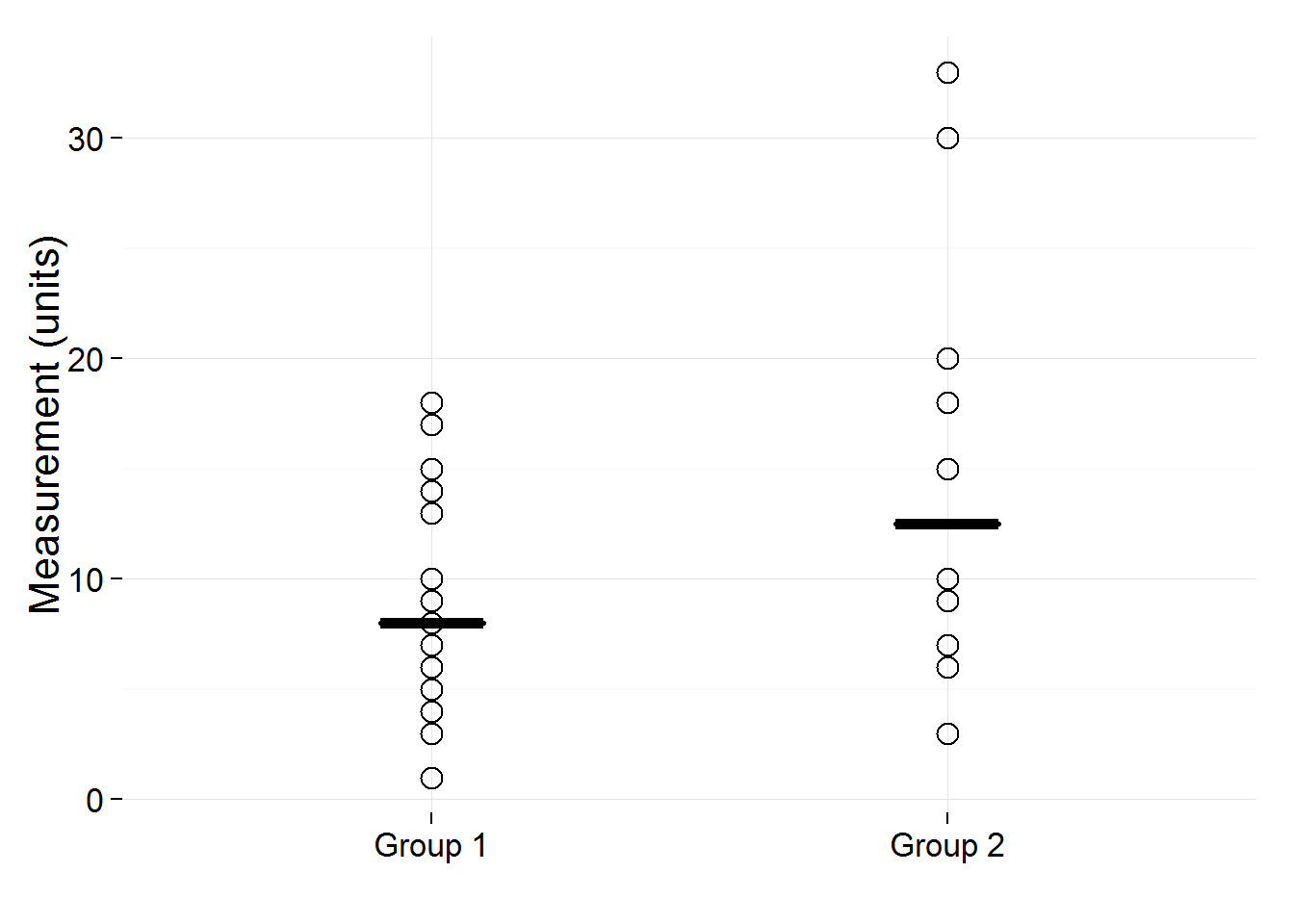Plotting groups 1, 2, and 3, the only thing that changes is the subsetting method:

# subset groups 1, 2 & 3
independent_data_long_groups_1_2_3 <-  independent_data_long %>%
filter(group %in% c("Group 1", "Group 2", "Group 3"))
# plot
ggplot(independent_data_long_groups_1_2_3, aes(group, as.numeric(value))) +
geom_point(shape = 1, size = 4) +
stat_summary(fun.y = median, fun.ymin = median, fun.ymax = median, geom = "crossbar", width = 0.2, size = 1) +
xlab("") +
ylab("Measurement (units)") +
theme_minimal(base_size = 16)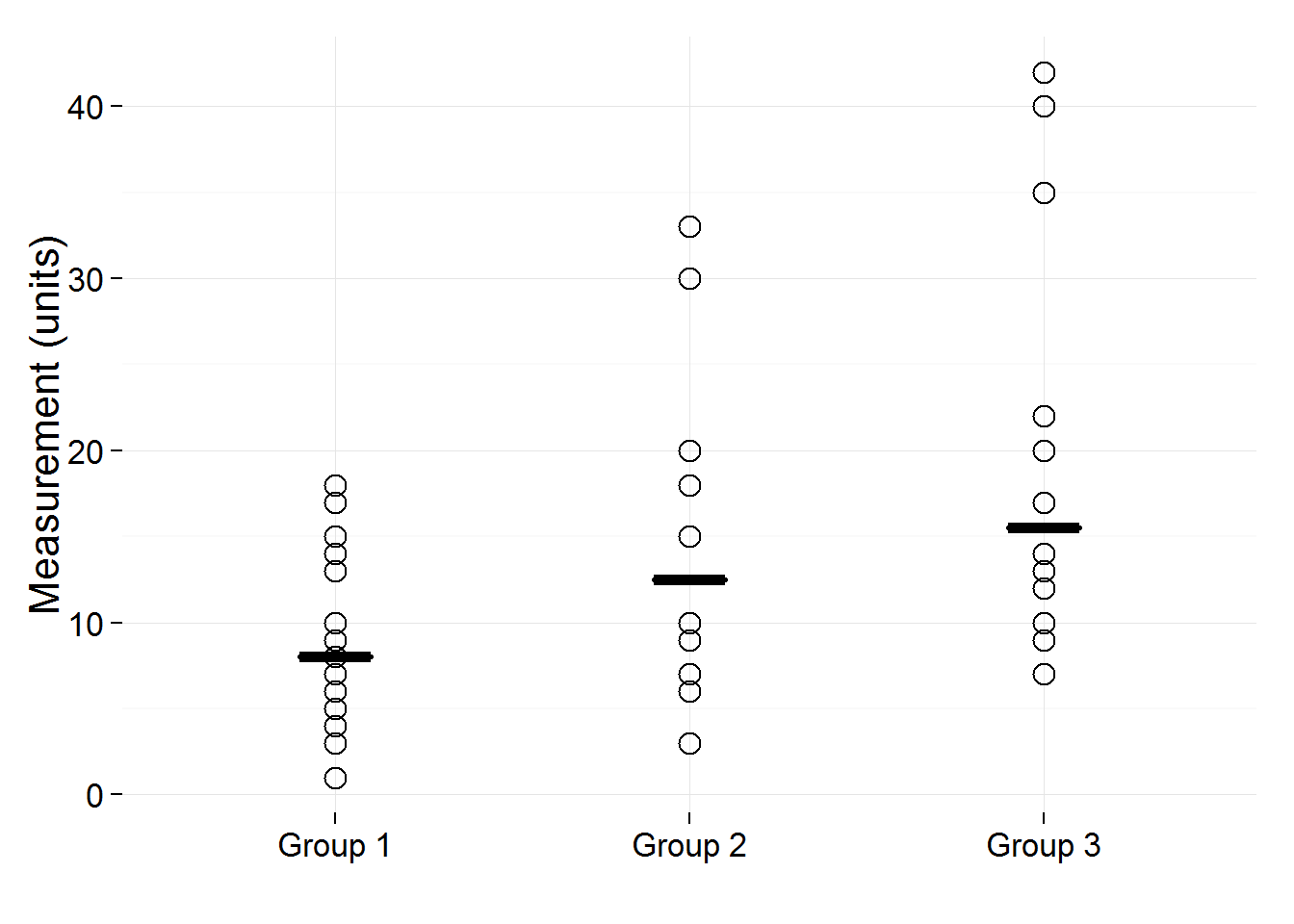Plotting groups 1 to 4:

# groups 1, 2, 3, & 4
independent_data_long_groups_1_2_3_4 <-  independent_data_long %>%
filter(group %in% c("Group 1", "Group 2", "Group 3", "Group 4"))
# plot
ggplot(independent_data_long_groups_1_2_3_4, aes(group, as.numeric(value))) +
geom_point(shape = 1, size = 4) +
stat_summary(fun.y = median, fun.ymin = median, fun.ymax = median, geom = "crossbar", width = 0.2, size = 1) +
xlab("") +
ylab("Measurement (units)") +
theme_minimal(base_size = 16)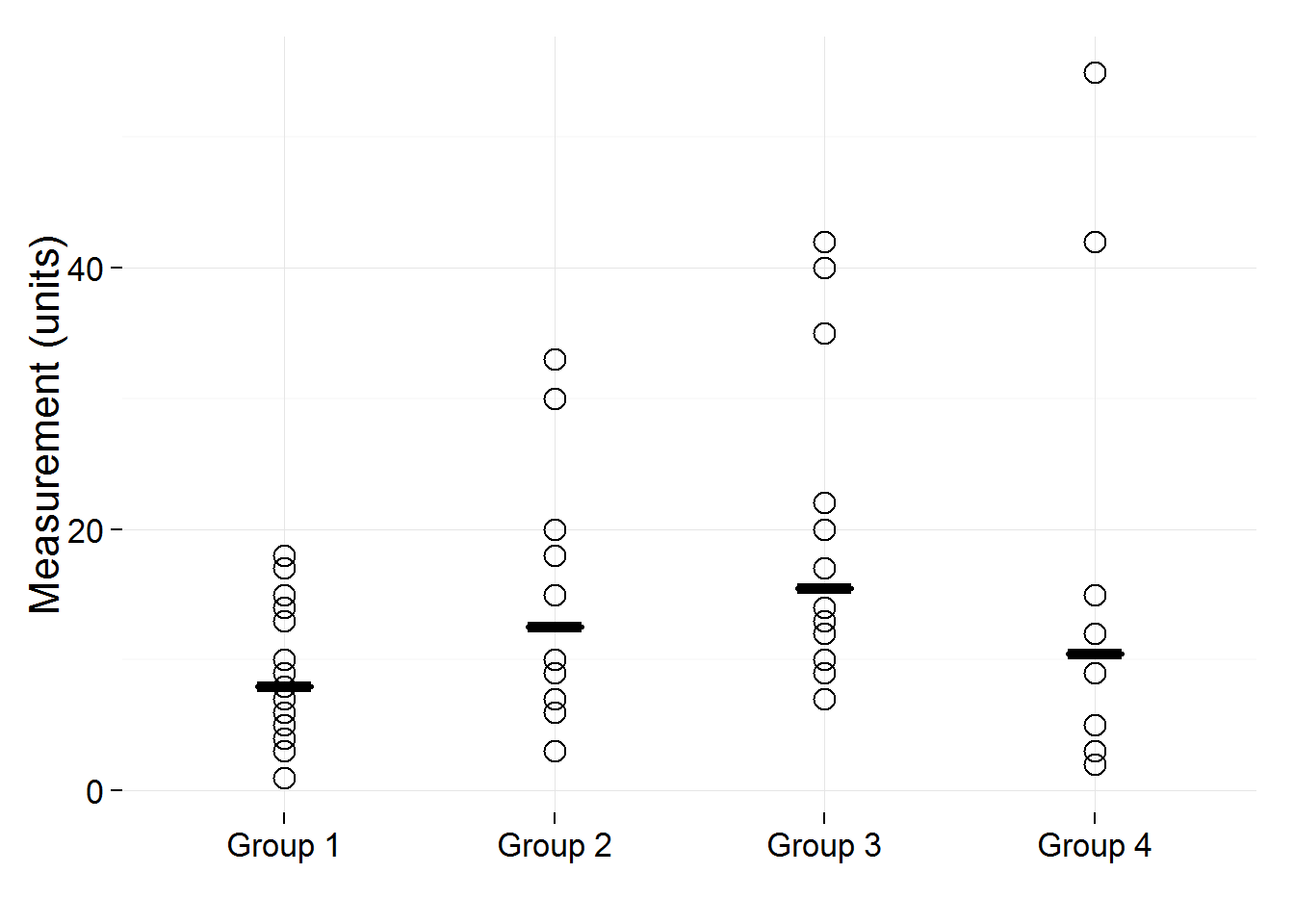And finally plotting all five groups, no subsetting required:

# all five groups
ggplot(independent_data_long, aes(group, as.numeric(value))) +
geom_point(shape = 1, size = 4) +
stat_summary(fun.y = median, fun.ymin = median, fun.ymax = median, geom = "crossbar", width = 0.2, size = 1) +
xlab("") +
ylab("Measurement (units)") +
theme_minimal(base_size = 16)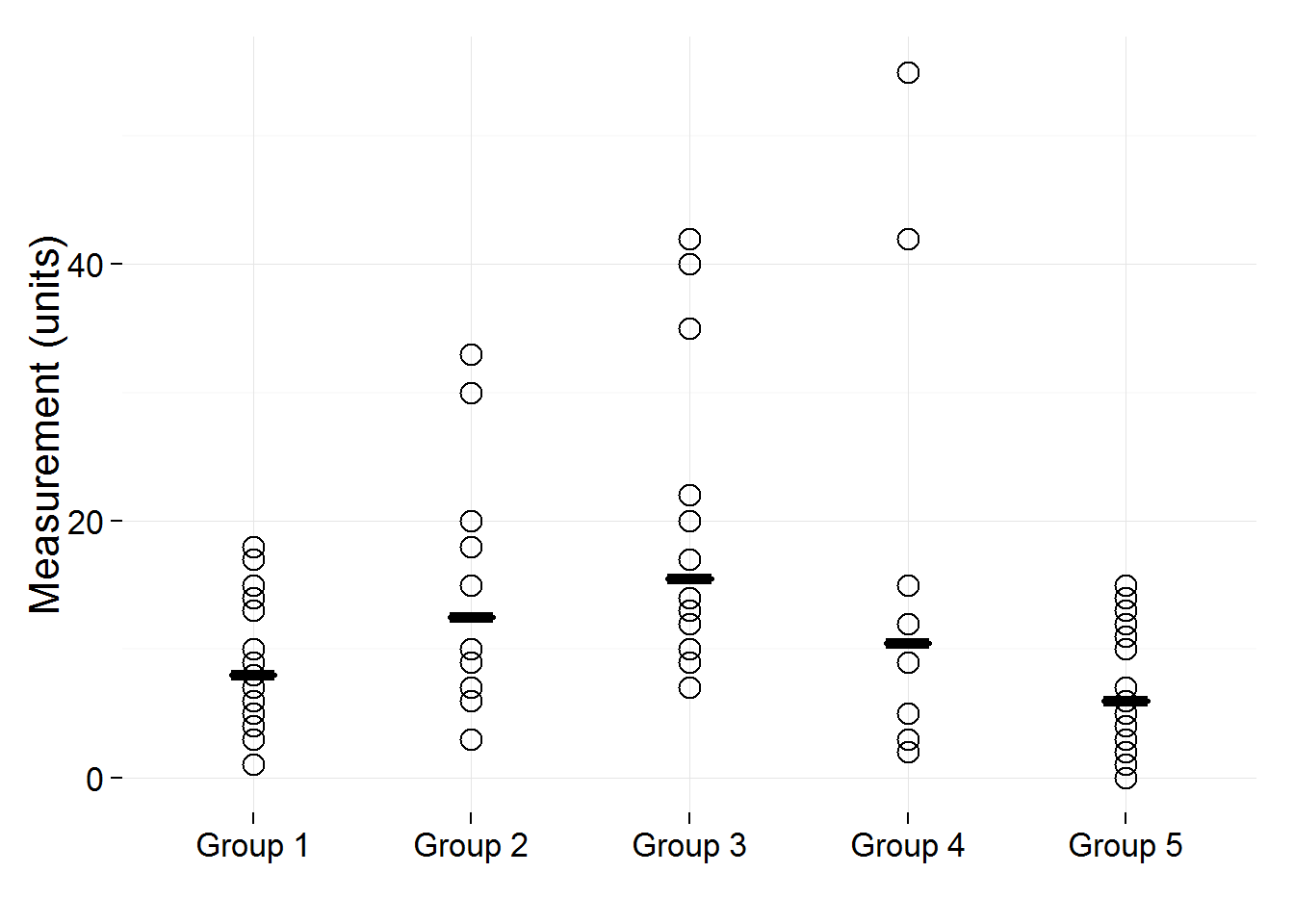## Independent data, points jittered

Use the code chunks below to create scatterplots for independent data in two to five groups, when there are overlapping points in at least one group. Independent data means that the variable of interest is measured one time in each subject, and subjects are not related to each other. If your data do not meet this criteria, see the code chunks below for paired or non-independent data. Overlapping points means that two subjects have values that are so close that they will overlap on the plot, and you will not be able to see both points clearly. Adjust the width and height values in the position_jitter function to refine the jitter settings for the points so that they do no overlap. Note that I’ve set a jitter height value, which is not part of the Excel plots, but I think is quite effective at separating points.

First we get the data from the Excel spreadsheet:

library(readxl)
# read in data from PLOS Biology article supplementary materials
independent_data_j <- read_excel("journal.pbio.1002128.s002.XLSX", sheet = 2)
# subset data from the 'points jittered' sheet
independent_data_j <- independent_data_j[16:115,2:3]
# group numbers are not given in the spreadsheet, so we'll add them
independent_data_j$Groups <- c(rep(1, 20), rep(2, 20), rep(3, 20), rep(4, 20), rep(5, 20)) # assign column names names(independent_data_j) <- c("Subject ID", "Measurement", "Group") The data are already in a nice tidy long format, with Group Name in one column and Measurement Values in another column, so we don’t need to reshape them. We can go directly to plotting them, first two groups, then three, then four, then all five groups. Once again the only thing that varies is how we subset the original data. # plot library(ggplot2) library(dplyr) # groups 1 & 2 independent_data_j_groups_1_and_2 <- independent_data_j %>% filter(Group %in% 1:2) # plot ggplot(independent_data_j_groups_1_and_2, aes(as.factor(Group), as.numeric(Measurement))) + geom_jitter(shape = 1, size = 4, position=position_jitter(width = 0.2, height = 0.2)) + stat_summary(fun.y = median, fun.ymin = median, fun.ymax = median, geom = "crossbar", width = 0.2, size = 1) + xlab("") + ylab("Measurement (units)") + theme_minimal(base_size = 16)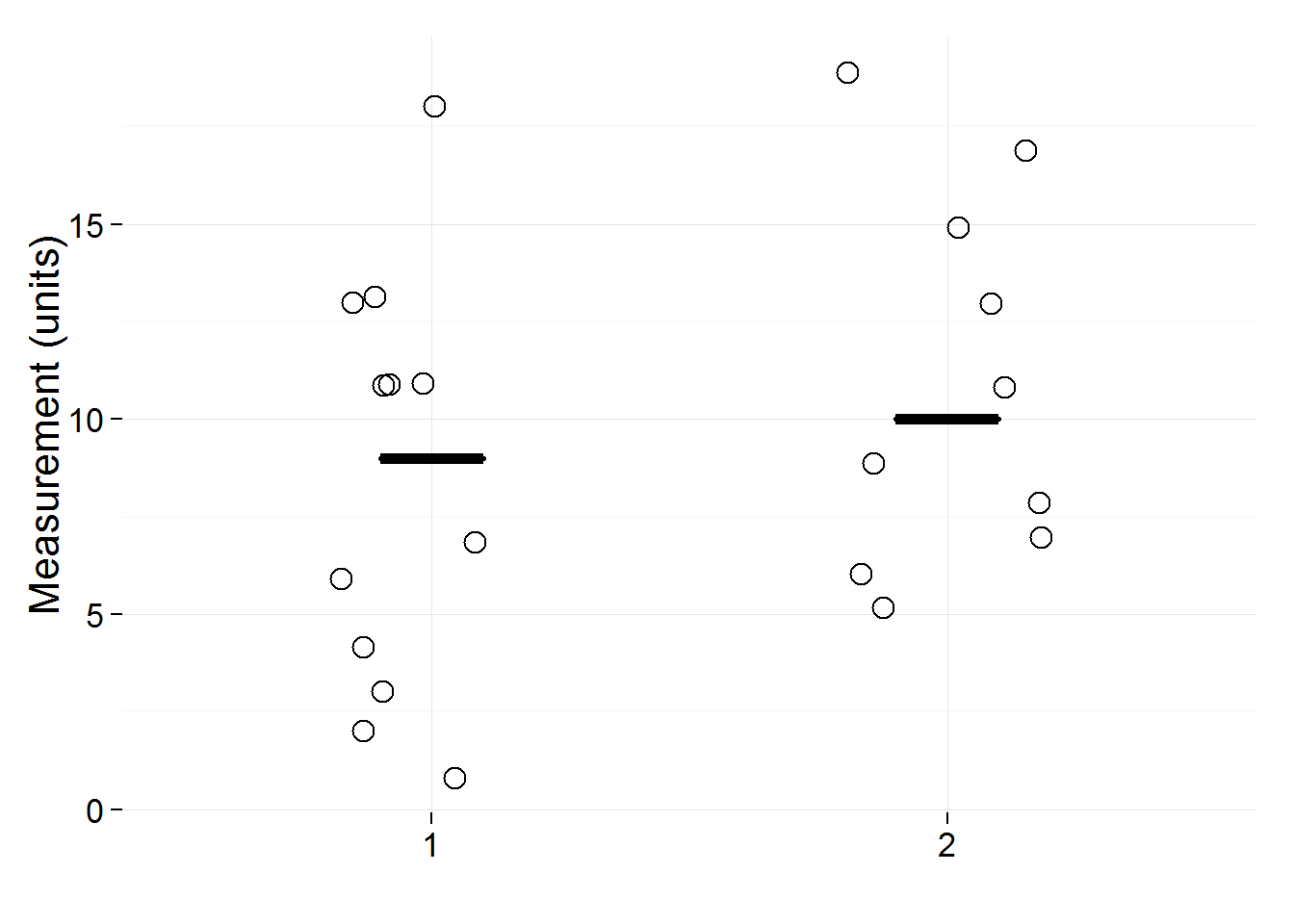# groups 1, 2 & 3 independent_data_j_groups_1_2_3 <- independent_data_j %>% filter(Group %in% 1:3) # plot ggplot(independent_data_j_groups_1_2_3, aes(as.factor(Group), as.numeric(Measurement))) + geom_jitter(shape = 1, size = 4, position=position_jitter(width = 0.2, height = 0.2)) + stat_summary(fun.y = median, fun.ymin = median, fun.ymax = median, geom = "crossbar", width = 0.2, size = 1) + xlab("") + ylab("Measurement (units)") + theme_minimal(base_size = 16)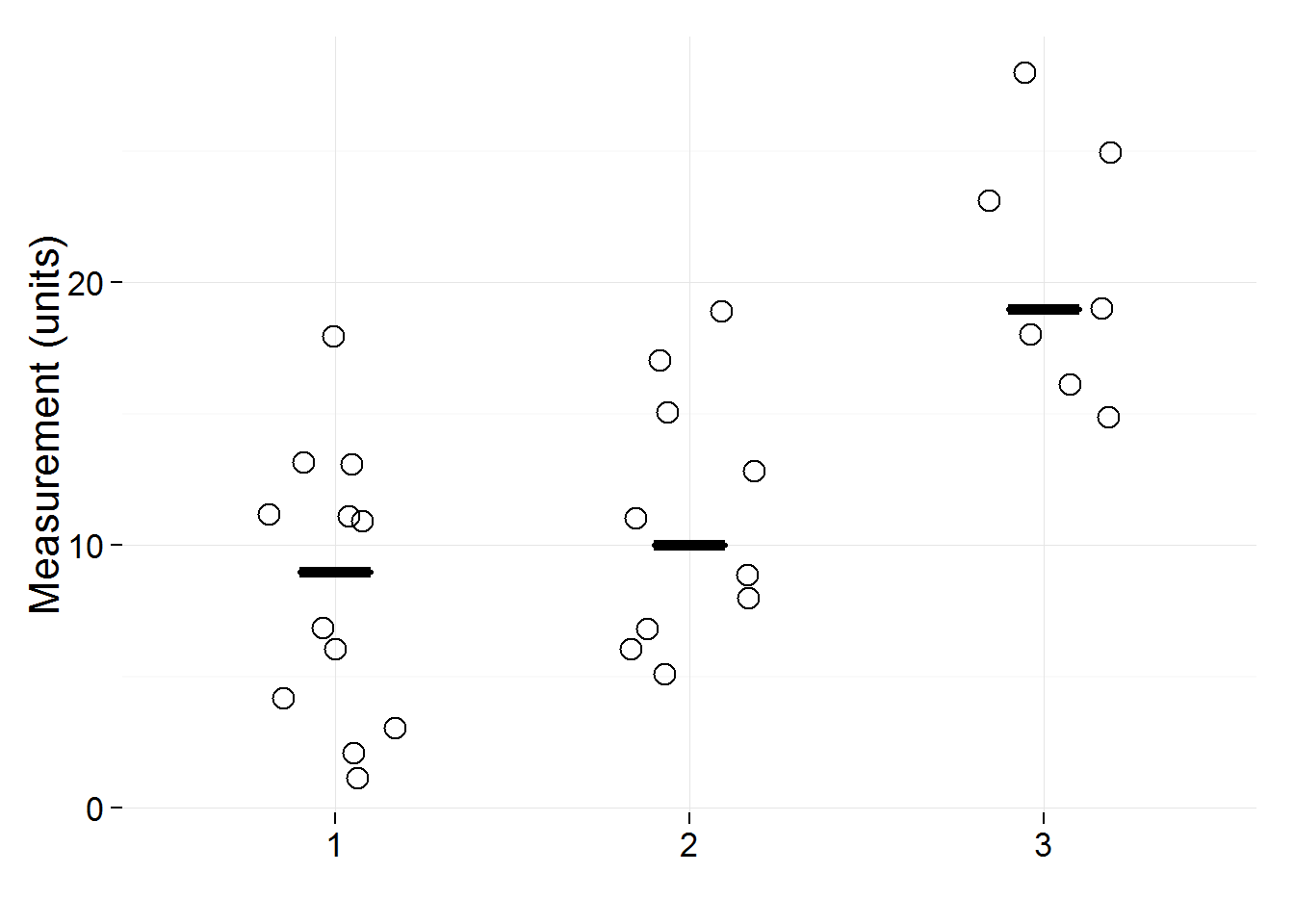# groups 1, 2, 3, & 4 independent_data_j_groups_1_2_3_4 <- independent_data_j %>% filter(Group %in% 1:4) # plot ggplot(independent_data_j_groups_1_2_3_4, aes(as.factor(Group), as.numeric(Measurement))) + geom_jitter(shape = 1, size = 4, position=position_jitter(width = 0.2, height = 0.2)) + stat_summary(fun.y = median, fun.ymin = median, fun.ymax = median, geom = "crossbar", width = 0.2, size = 1) + xlab("") + ylab("Measurement (units)") + theme_minimal(base_size = 16)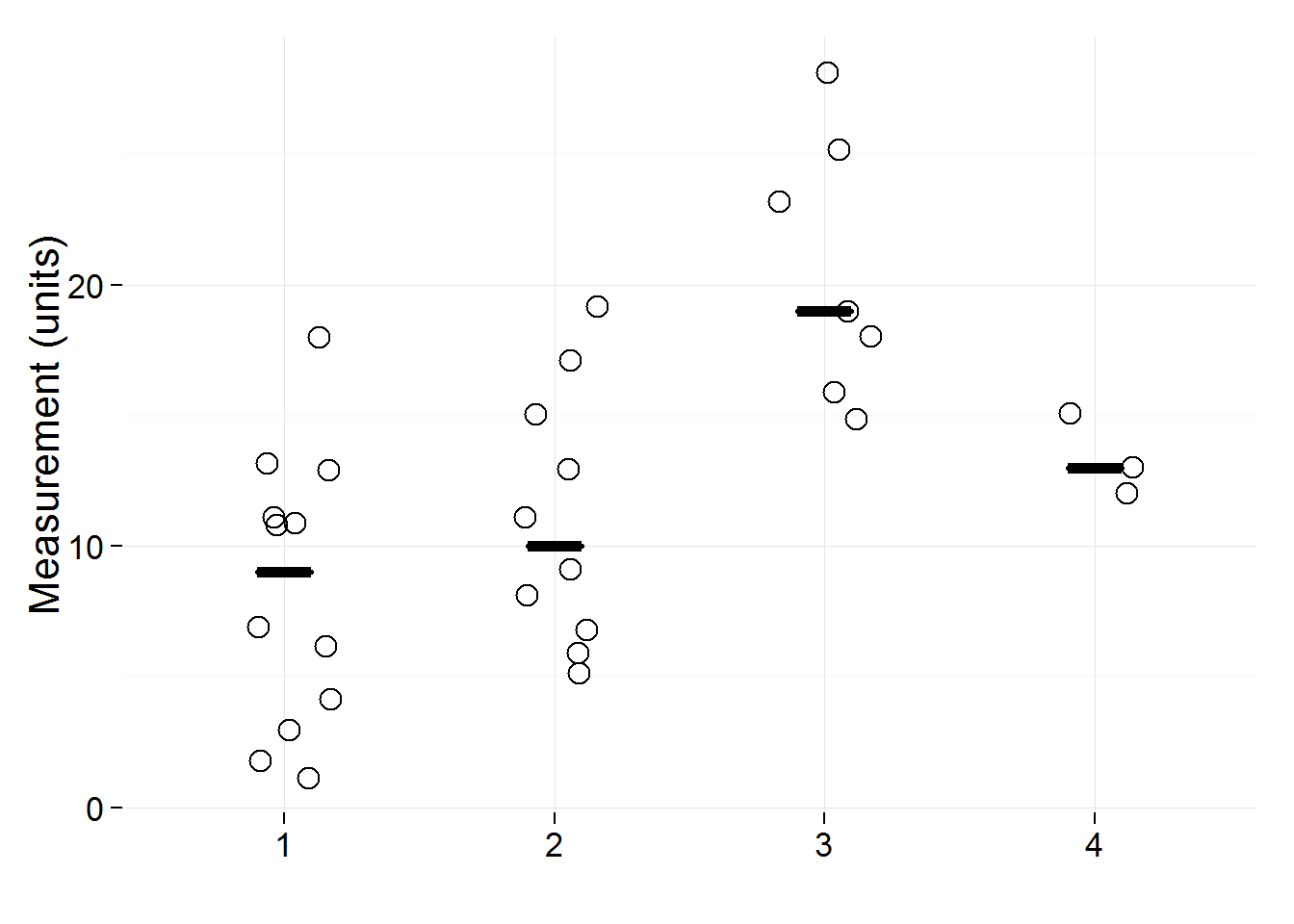# all five groups ggplot(independent_data_j, aes(as.factor(Group), as.numeric(Measurement))) + geom_jitter(shape = 1, size = 4, position=position_jitter(width = 0.2, height = 0.2)) + stat_summary(fun.y = median, fun.ymin = median, fun.ymax = median, geom = "crossbar", width = 0.2, size = 1) + xlab("") + ylab("Measurement (units)") + theme_minimal(base_size = 16)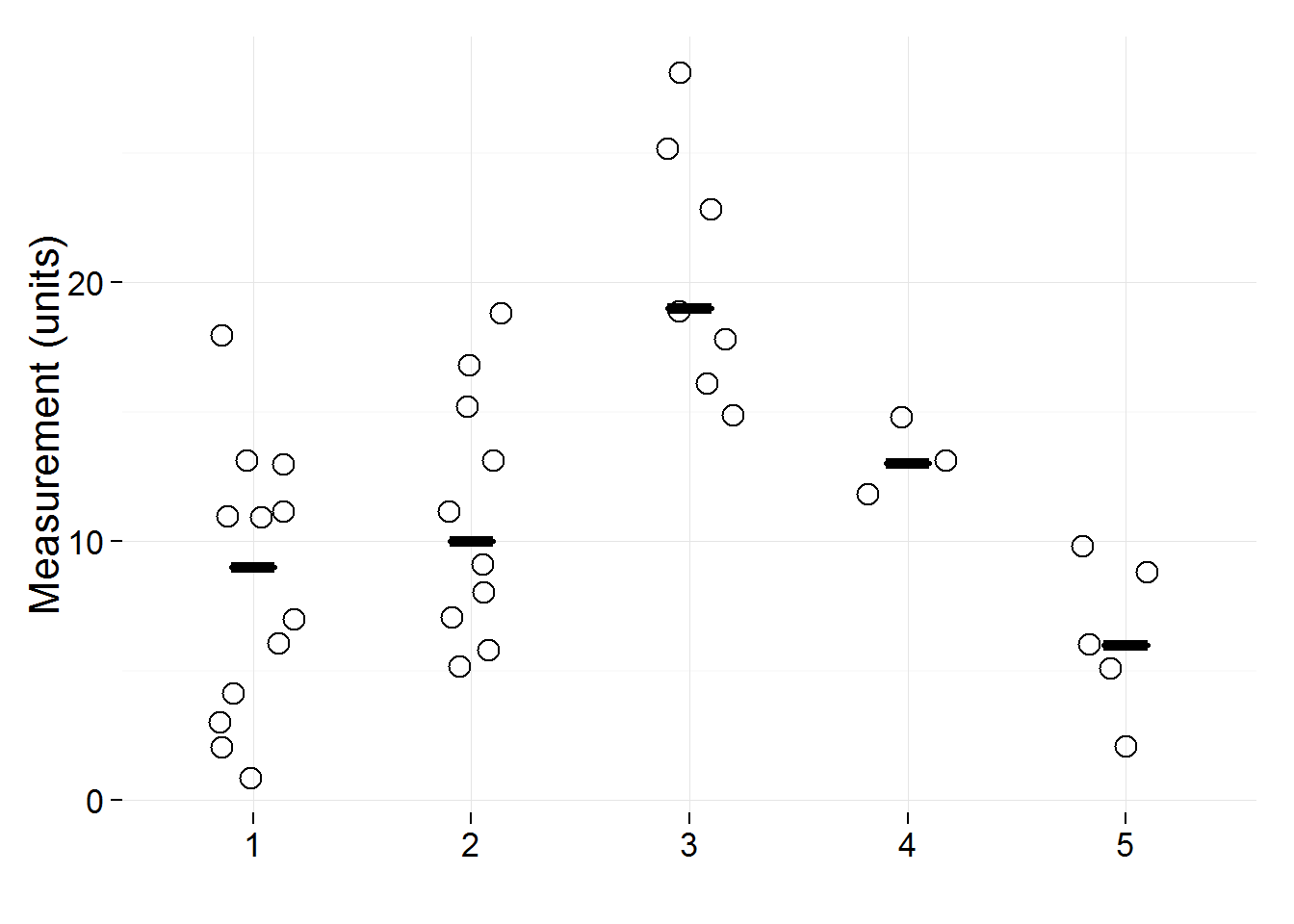## Paired or Non-independent Data: 1 Group, 2 Conditions Use these chunks to create scatterplots for paired or matched data (2 conditions) in one group of subjects. Paired data are when you measure the variable of interest more than one time in each participant. Matched data are when participants in groups 1 and 2 are matched for important characteristics. If your data are independent, please see the chunks above for Independent Data. The short horizontal black line in the “Difference in Measurement” graph shows the median difference. Medians for each condition are not shown, and should not be calculated. Unlike means, medians are not additive. The median difference does not equal the difference in the medians. Open circles and black lines connecting the circles show paired measurements for each participant or observation. library(readxl) # read in data from PLOS Biology article supplementary materials One_group_two_conditions <- read_excel("journal.pbio.1002128.s003.XLS", sheet = 1) # subset data from the 'points jittered' sheet One_group_two_conditions <- One_group_two_conditions[12:23,1:3] # assign column names names(One_group_two_conditions) <- c("Subject ID", "Condition 1 Name", "Condition 2 Name") One_group_two_conditions$difference <- as.numeric(One_group_two_conditions$Condition 2 Name) - as.numeric(One_group_two_conditions$Condition 1 Name)

The data in the Excel sheet are in an untidy wide format, so let’s convert them to a tidy long format:

# reshape for plotting
library(tidyr)
One_group_two_conditions_long <- gather(One_group_two_conditions, group, value, Condition 1 Name:Condition 2 Name, -Subject ID, -difference, convert = TRUE)

Now we can plot:

# plot
library(ggplot2)
library(gridExtra)

g1 <- ggplot(One_group_two_conditions_long, aes(group, as.numeric(value), group = Subject ID)) +
geom_point(shape = 1, size = 4) +
geom_line() +
xlab("") +
ylab("Measurement (units)") +
theme_minimal(base_size = 16) +
theme(axis.text.x = element_text(angle = 90, vjust = 0.5, hjust = 1))

# differences
g2 <- ggplot(One_group_two_conditions_long, aes(x = 1, y = difference)) +
geom_point(shape = 1, size = 4) +
stat_summary(fun.y = median, fun.ymin = median, fun.ymax = median, geom = "crossbar", width = 0.001, size = 1) +
xlab("") +
ylab("Difference in Measurement (units)") +
theme_minimal(base_size = 16) +
scale_x_continuous(breaks = NULL) +
coord_fixed(ratio = 0.0005)

# combine the two plots
grid.arrange(g1, g2, ncol = 2)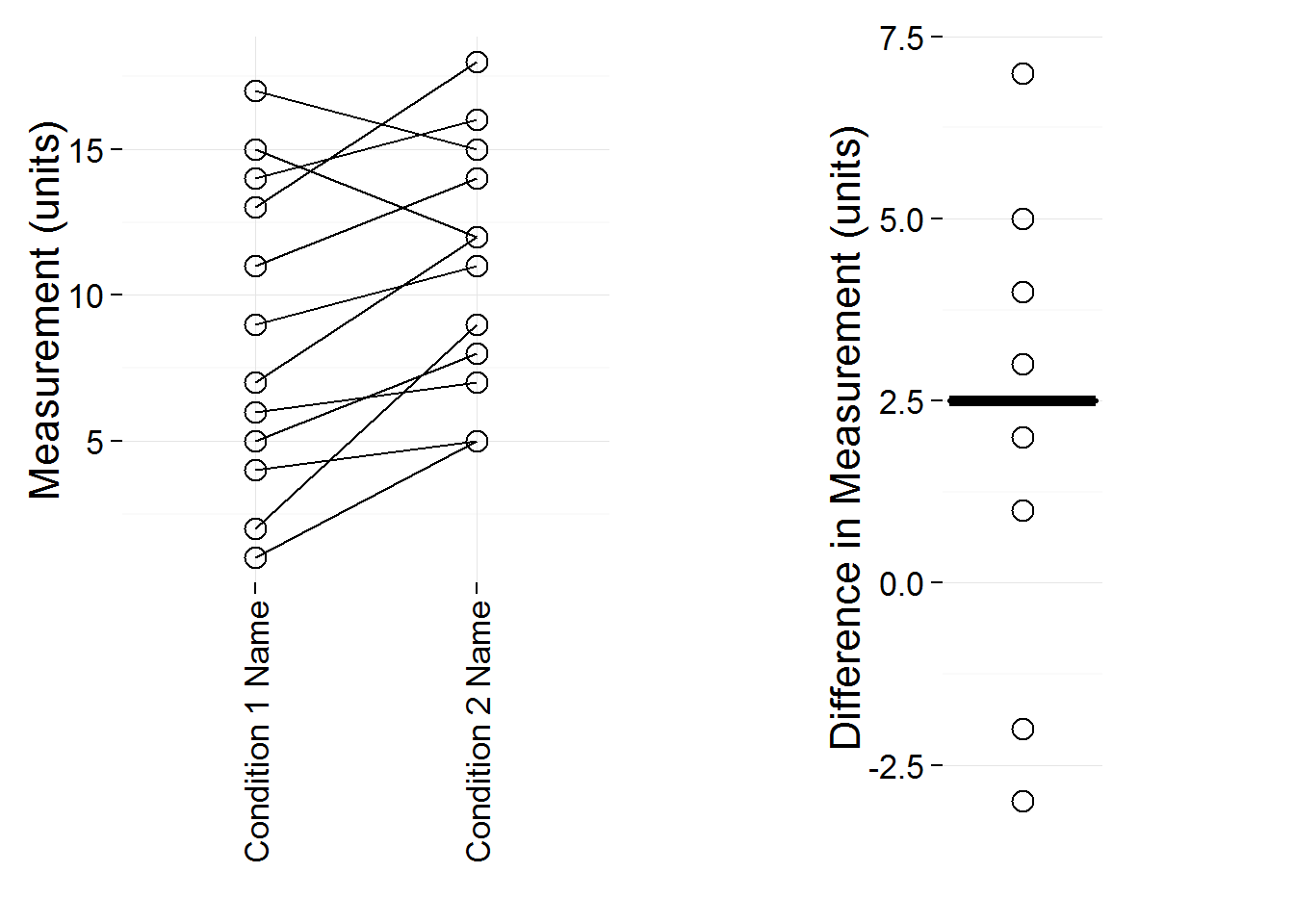## Paired or Non-independent Data: 2 Groups, 2 Conditions

library(readxl)
# read in data from PLOS Biology article supplementary materials
Two_groups_two_conditions <- read_excel("journal.pbio.1002128.s003.XLS", sheet = 2)
# subset data from the 'points jittered' sheet
Two_groups_two_conditions <- Two_groups_two_conditions[12:41,2:5]
# assign group names
Two_groups_two_conditions$group <- c(rep("Group 1 Name", 15), rep("Group 2 Name", 15)) names(Two_groups_two_conditions) <- c("Condition 1 Name A", "Condition 2 Name A", "Condition 1 Name B", "Condition 2 Name B") The data in the Excel sheet are in an unusual structure, so a few steps for reshaping into a tidy form are needed. Here’s how we can tidy them and how they look after being tidied: # convert to simple long form Two_groups_two_conditions[,1] <- unlist(c(Two_groups_two_conditions[1:15,1], Two_groups_two_conditions[16:30,3])) Two_groups_two_conditions[,2] <- unlist(c(Two_groups_two_conditions[1:15,2], Two_groups_two_conditions[16:30,4])) # drop unneeded columns Two_groups_two_conditions <- Two_groups_two_conditions[,c(1:2, 5)] # assign column names names(Two_groups_two_conditions) <- c("Condition 1", "Condition 2", "Group") Two_groups_two_conditions$Subject ID <- 1:30
# compute differences
Two_groups_two_conditions$difference <- as.numeric(Two_groups_two_conditions$Condition 2)  - as.numeric(Two_groups_two_conditions\$Condition 1)

# convert to long again
library(tidyr)
Two_groups_two_conditions_long <- gather(Two_groups_two_conditions, condition, value, c(Condition 1, Condition 2), convert = TRUE)
kable(Two_groups_two_conditions_long)
Group Subject ID difference condition value
Group 1 Name 1 8 Condition 1 5.000000
Group 1 Name 2 4 Condition 1 1.000000
Group 1 Name 3 5 Condition 1 7.000000
Group 1 Name 4 2 Condition 1 9.000000
Group 1 Name 5 7 Condition 1 2.000000
Group 1 Name 6 -1 Condition 1 6.000000
Group 1 Name 7 1 Condition 1 4.000000
Group 1 Name 8 3 Condition 1 11.000000
Group 1 Name 9 -2 Condition 1 14.000000
Group 1 Name 10 6 Condition 1 13.000000
Group 1 Name 11 NA Condition 1 NA
Group 1 Name 12 NA Condition 1 NA
Group 1 Name 13 NA Condition 1 NA
Group 1 Name 14 NA Condition 1 NA
Group 1 Name 15 NA Condition 1 NA
Group 2 Name 16 -2 Condition 1 20.000000
Group 2 Name 17 -4 Condition 1 13.000000
Group 2 Name 18 1 Condition 1 15.000000
Group 2 Name 19 5 Condition 1 8.000000
Group 2 Name 20 2 Condition 1 3.000000
Group 2 Name 21 1 Condition 1 7.000000
Group 2 Name 22 -7 Condition 1 14.000000
Group 2 Name 23 0 Condition 1 12.000000
Group 2 Name 24 3 Condition 1 11.000000
Group 2 Name 25 1 Condition 1 9.000000
Group 2 Name 26 NA Condition 1 NA
Group 2 Name 27 NA Condition 1 NA
Group 2 Name 28 NA Condition 1 NA
Group 2 Name 29 NA Condition 1 NA
Group 2 Name 30 NA Condition 1 NA
Group 1 Name 1 8 Condition 2 13.000000
Group 1 Name 2 4 Condition 2 5.000000
Group 1 Name 3 5 Condition 2 12.000000
Group 1 Name 4 2 Condition 2 11.000000
Group 1 Name 5 7 Condition 2 9.000000
Group 1 Name 6 -1 Condition 2 5.000000
Group 1 Name 7 1 Condition 2 5.000000
Group 1 Name 8 3 Condition 2 14.000000
Group 1 Name 9 -2 Condition 2 12.000000
Group 1 Name 10 6 Condition 2 19.000000
Group 1 Name 11 NA Condition 2 NA
Group 1 Name 12 NA Condition 2 NA
Group 1 Name 13 NA Condition 2 NA
Group 1 Name 14 NA Condition 2 NA
Group 1 Name 15 NA Condition 2 NA
Group 2 Name 16 -2 Condition 2 18.000000
Group 2 Name 17 -4 Condition 2 9.000000
Group 2 Name 18 1 Condition 2 16.000000
Group 2 Name 19 5 Condition 2 13.000000
Group 2 Name 20 2 Condition 2 5.000000
Group 2 Name 21 1 Condition 2 8.000000
Group 2 Name 22 -7 Condition 2 7.000000
Group 2 Name 23 0 Condition 2 12.000000
Group 2 Name 24 3 Condition 2 14.000000
Group 2 Name 25 1 Condition 2 10.000000
Group 2 Name 26 NA Condition 2 NA
Group 2 Name 27 NA Condition 2 NA
Group 2 Name 28 NA Condition 2 NA
Group 2 Name 29 NA Condition 2 NA
Group 2 Name 30 NA Condition 2 NA

Now we can plot:

# plot
library(ggplot2)
g1 <- ggplot(Two_groups_two_conditions_long, aes(condition, as.numeric(value), group = Subject ID)) +
geom_point(size = 4, shape = 1) +
geom_line() +
xlab("") +
ylab("Measurement (units)") +
theme_minimal(base_size = 16) +
facet_grid(~Group) +
theme(axis.text.x = element_text(angle = 90, vjust = 0.5, hjust=1))

# difference
g2 <-  ggplot(Two_groups_two_conditions_long, aes(Group, difference)) +
geom_point(size = 4, shape = 1) +
stat_summary(fun.y = median, fun.ymin = median, fun.ymax = median, geom = "crossbar", width = 0.3) +
xlab("") +
ylab("Difference in Measurement (units)") +
theme_minimal(base_size = 16)  +
coord_fixed(ratio = 0.15)

# combine the two plots
grid.arrange(g1, g2, ncol = 2)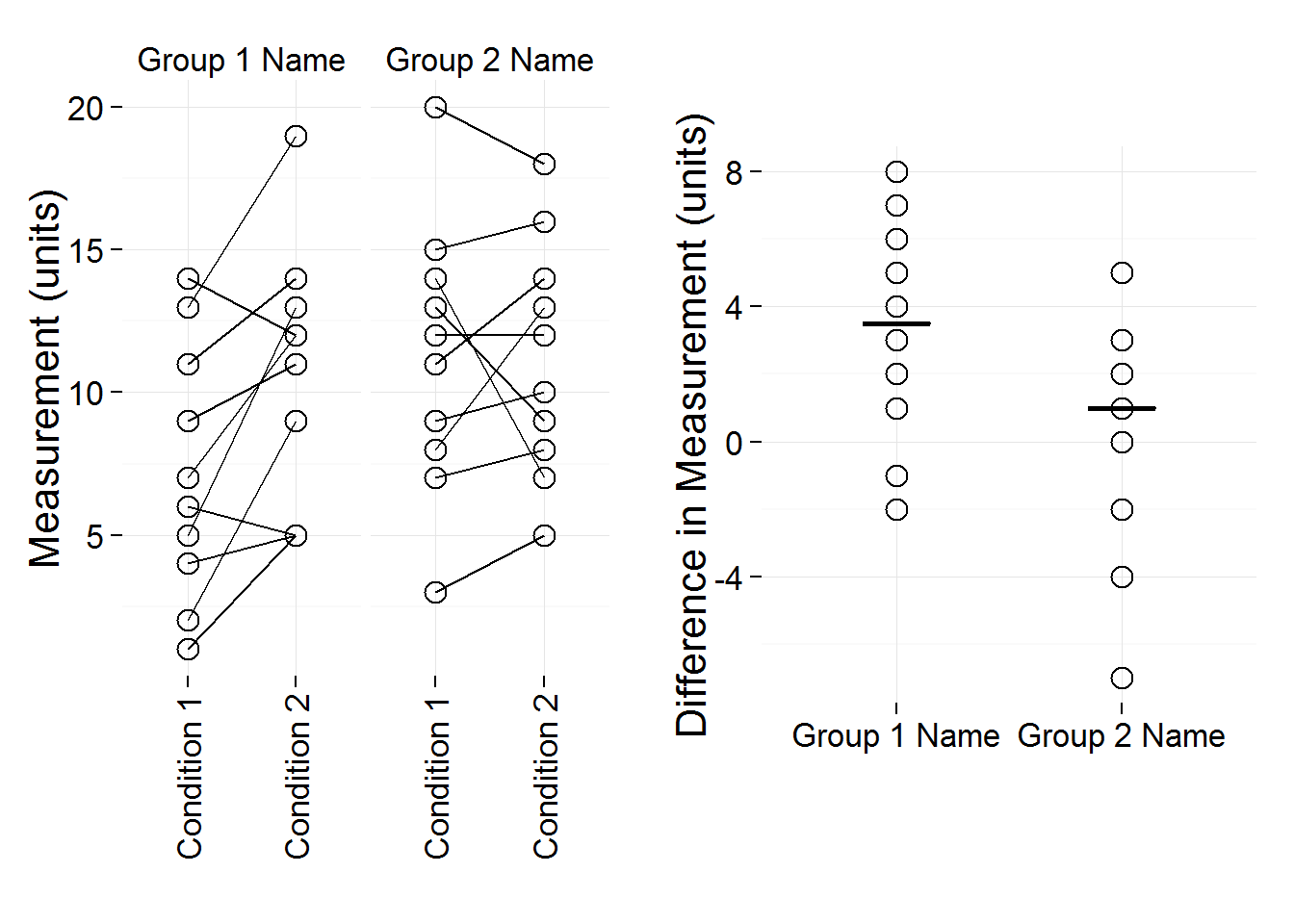Here’s a summary of the computational environment the these plots were produced in. If you want to reproduce the plots here you’ll need a similar version of R and the packages listed below:

sessionInfo()
## R version 3.2.0 alpha (2015-03-25 r68091)
## Platform: x86_64-w64-mingw32/x64 (64-bit)
## Running under: Windows 7 x64 (build 7601) Service Pack 1
##
## locale:
##  LC_COLLATE=English_United States.1252
##  LC_CTYPE=English_United States.1252
##  LC_MONETARY=English_United States.1252
##  LC_NUMERIC=C
##  LC_TIME=English_United States.1252
##
## attached base packages:
##  grid      stats     graphics  grDevices utils     datasets  methods
##  base
##
## other attached packages:
##  gridExtra_0.9.1 dplyr_0.4.1     ggplot2_1.0.1   tidyr_0.2.0
##  labeling_0.3     stringi_0.4-1    scales_0.2.4     proto_0.3-10